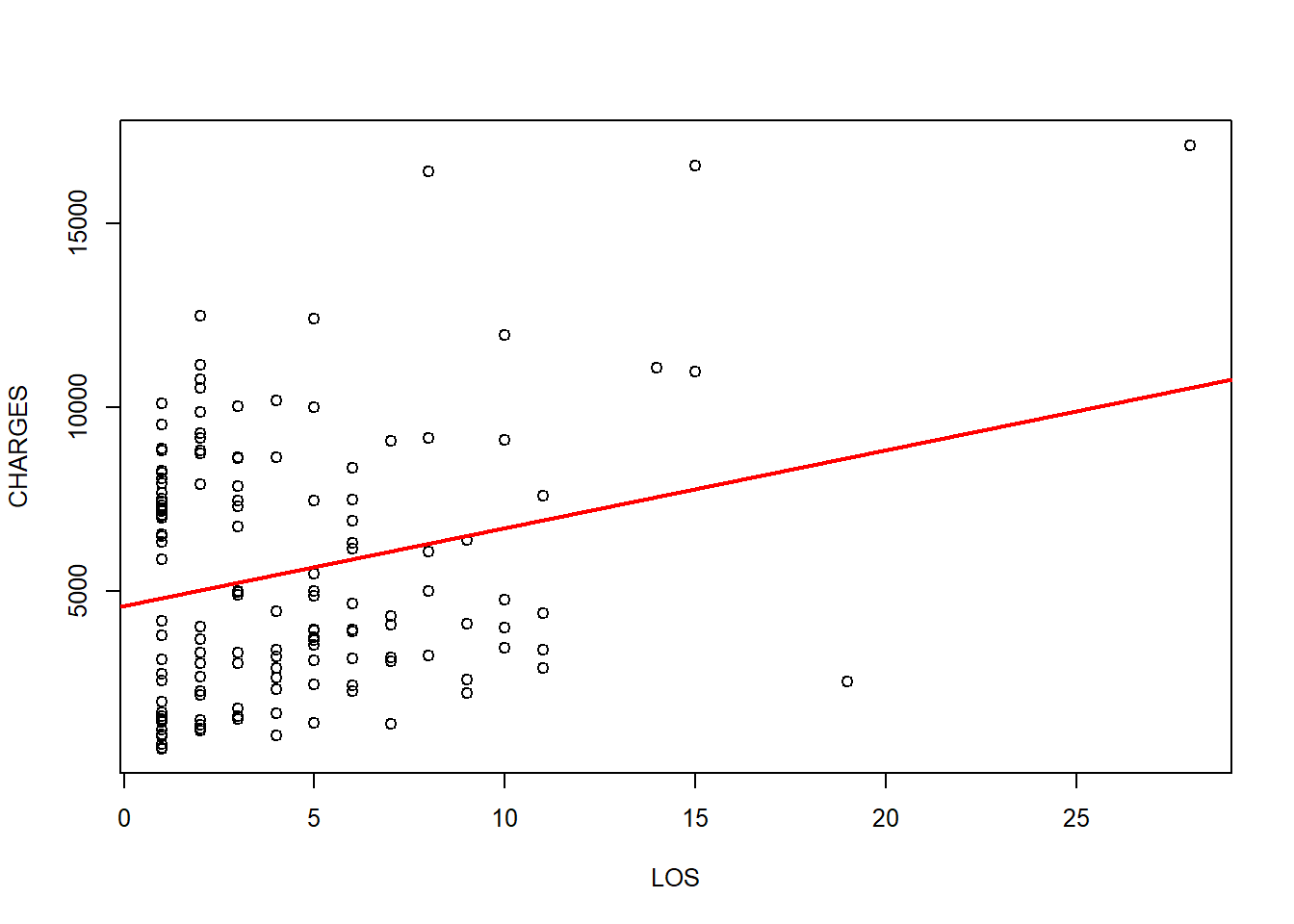# 1 Motivation

In previous the previous Chapter 6, Chapter 7, and Chapter 8, we covered some classification methods that use mathematical formalism to address everyday life prediction problems. In this chapter, we will focus on specific model-based statistical methods providing forecasting and classification functionality. Specifically, we will (1) demonstrate the predictive power of multiple linear regression, (2) show the foundation of regression trees and model trees, and (3) examine two complementary case-studies (Baseball Players and Heart Attack).

It may be helpful to first review Chapter 4 (Linear Algebra/Matrix Manipulations) and Chapter 6 (Introduction to Machine Learning).

# 2 Understanding Regression

Regression represents a model of a relationship between a dependent variable (value to be predicted) and a group of independent variables (predictors or features), see Chapter 6. We assume the relationships between the outcome dependent variable and the independent variables is linear.

## 2.1 Simple linear regression

First review the material in Chapter 4: Linear Algebra & Matrix Computing.

The straightforward case of regression is simple linear regression, which involves a single predictor. $y=a+bx.$

This formula would be familiar, as we showed examples in previous chapters. In this slope-intercept formula, a is the model intercept and b is the model slope. Thus, simple linear regression may be expressed as a bivariate equation. If we know a and b, for any given x we can estimate, or predict, y via the regression formula. If we plot x against y in a 2D coordinate system, where the two variables are exactly linearly related, the results will be a straight line.

However, this is the ideal case. Bivariate scatterplots using real world data may show patterns that are not necessarily precisely linear, see Chapter 2. Let’s look at a bivariate scatterplot and try to fit a simple linear regression line using two variables, e.g., hospital charges or CHARGES as a dependent variable, and length of stay in the hospital or LOS as an independent predictor. The data is available in the DSPA Data folder as CaseStudy12_AdultsHeartAttack_Data. We can remove the pair of observations with missing values using the command heart_attack<-heart_attack[complete.cases(heart_attack), ].

heart_attack<-read.csv("https://umich.instructure.com/files/1644953/download?download_frd=1", stringsAsFactors = F)
heart_attack$CHARGES<-as.numeric(heart_attack$CHARGES)
heart_attack<-heart_attack[complete.cases(heart_attack), ]

fit1<-lm(CHARGES~LOS, data=heart_attack)
par(cex=.8)
plot(heart_attack$LOS, heart_attack$CHARGES, xlab="LOS", ylab = "CHARGES")
abline(fit1, lwd=2, col="red")As expected, longer hospital stays are expected to be associated with higher medical costs, or hospital charges. The scatterplot shows dots for each pair of observed measurements ($$x=LOS$$ and $$y=CHARGES$$), and an increasing linear trend.

The estimated expression for this regression line is: $\hat{y}=4582.70+212.29\times x$ or equivalently $CHARGES=4582.70+212.29\times LOS$ Once the linear model is fit, i.e., its coefficients are estimated, we can make predictions using this explicit regression model. Assume we have a patient that spent 10 days in hospital, then we have LOS=10. The predicted charge is likely to be $$\ 4582.70 + \ 212.29 \times 10= \ 6705.6$$. Plugging x into the expression equation automatically gives us an estimated value of the outcome y. This chapter of the Probability and statistics EBook provides an introduction to linear modeling.

## 2.2 Ordinary least squares estimation

How did we get the estimated expression? The most common estimating method in statistics is ordinary least squares (OLS). OLS estimators are obtained by minimizing sum of the squared errors - that is the sum of squared vertical distance from each dot on the scatter plot to the regression line.